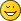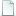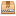Static 5 bars linkage angles calculation

Static 5 bars linkage angles calculation
#1
Anyone knows how to calculate the angles of such a 5 bars linkage:Graphically it's relatively simple (intersection of the locus of a 4 bars linkage with the circle of the 5th bar), but analytically it's another story...
The problem surfaced when modeling top support of the boom of 42042 crane B-model that uses this kind of structureI'll probably use a successive approximation method, but if somone has a better way...
Re: Static 5 bars linkage angles calculation
#2
You can reproduce it in some CAD software and read the angles.

I can do it for you next week if you want.

Then, I guess a bit of trigonometry could do the job also, but it will be a bit more painful.
Re: Static 5 bars linkage angles calculation
#4
Yes, with a good CAD software it should be possible...
But I will try to find a solution without using CAD software :-D

/Max
Re: Static 5 bars linkage angles calculation
#5
Thanks bothNote that I am more interested by the method than by the solution...
Re: Static 5 bars linkage angles calculation
#6
The graphical "solution"...Done with online version of GeoGebra.
Re: Static 5 bars linkage angles calculation
#7
I guess you can write the whole thing down as a simultaneous equation involving the various angles and radii (or x,y,z values). But I suspect there is no simple "rule of thumb" because, at a glance, it looks like you're dealing with a solution of greater than cubic complexity. And those only have closed form solutions in limited cases.

All the best,

Tim
Re: Static 5 bars linkage angles calculation
#8
You are right Tim, it's easy to get configuration that show 4 solutions, so equations may have no analytical solutions. Fiddling with Geogebra, I came to the kind of configuration below, where the 5th bar tip circle is more or less tangent to the locus of 4 bars linkage. Physically, with the play in joints, you get something no longer static, that can move in some range...Re: Static 5 bars linkage angles calculation
#9
...and it was finally a pointless question, I had to use some stretching anyway.
Re: Static 5 bars linkage angles calculation
#10
I still try to find a solution to solve this system mathematically, just because I'm interested in how to solve this problem. And I'm quite sure, that there must be at least one solution for this system, cause Grübler's equation results in 0, so it's a simple static system.

/Max
Re: Static 5 bars linkage angles calculation
#11
The way I normally do these is to just throw some basic triangle equations into Excel and use some macrobuttons to nudge the controlling beam angle in coarse/fine amounts until the "error" (between where the constraining beam top pin landed and where it ought to have landed) hits zero, or as close as 0.000001 since LEGO will flex a little anyway. Takes about 15 minutes to setup and 30 seconds to find the answer. Much faster than trying to solve equations properly, especially in my old age. Besides, that's what computers are forI was intrigued at the prospect of solving it analytically though. With the method I use, technically it would be a matter of taking the 1st order derivative of the "error" function and solving for zero, essentially finding the turning-point where the "error" graph is at a minimum. But I pasted the sub-equations I used from the spreadsheet into one giant equation and promptly found that it would be extremely difficult to differentiate (even using chain rule) let alone solve for zero. Most of the online derivative calculators choked at the length of the equation, but the GeoGebra site Philo used was at least able to graph it and also let me easily find the same control angle I got from Excel. I stopped at 6dp, but could easily have gotten more.

If anyone's interested (or wants a laugh) this was the equation that computes the "error" from the control angle x. Excuse the excess brackets, didn't want to introduce any errors as I substituted all the sub-equations into the outer equation. Differentiate and solve this for zero and the job's donef(x)=(sqrt((((((sin(x)*120)-(sin((acos((33600+(((sin(x)*120)^2)+((240-(cos(x)*120))^2)))/(400*sqrt(((sin(x)*120)^2)+((240-(cos(x)*120))^2))))+asin((sin(x)*120)/sqrt(((sin(x)*120)^2)+((240-(cos(x)*120))^2)))))*200))/2)+(sin((acos((33600+(((sin(x)*120)^2)+((240-(cos(x)*120))^2)))/(400*sqrt(((sin(x)*120)^2)+((240-(cos(x)*120))^2))))+asin((sin(x)*120)/sqrt(((sin(x)*120)^2)+((240-(cos(x)*120))^2)))))*200))^2)+(((((((cos((acos((33600+(((sin(x)*120)^2)+((240-(cos(x)*120))^2)))/(400*sqrt(((sin(x)*120)^2)+((240-(cos(x)*120))^2))))+asin((sin(x)*120)/sqrt(((sin(x)*120)^2)+((240-(cos(x)*120))^2)))))*200)-240)-(cos(x)*-120))/2)+(cos(x)*-120))+60)^2))-120)

Edit: actually, come to think of it, no derivative necessary. Just need to solve for zero. But I think I'll still stick to graphs or successive approximation!

Attached Filesbeams.ldr (Size: 980 bytes / Downloads: 0)
Re: Static 5 bars linkage angles calculation
#12
NiceRe: Static 5 bars linkage angles calculation
#13
Sure. It's quite an older copy of Excel, but if MS have done their job right it will hopefully load into a more modern version without formatting issues!

It's pretty messy in there as I tend to throw these things together hastily, so I added a few comments in the sheet to help explain.

One fixed beam, one control beam, two follower beams, modelled with simple triangle math and this 4-beam structure free to move between two limits. Fifth constraining beam then added to fixed beam and projected through target destination point on top follower beam, calculating the error of how much the final pin missed its target by.

Attached Filesbeams.zip (Size: 67.23 KB / Downloads: 0)
Re: Static 5 bars linkage angles calculation
#3
That's a complex task. I will see, if I can find an adequate way for solving, tomorrow. ;-)

/Max
« Next Oldest | Next Newest »

Forum Jump:

Users browsing this thread: 1 Guest(s)